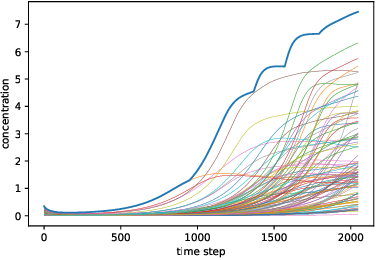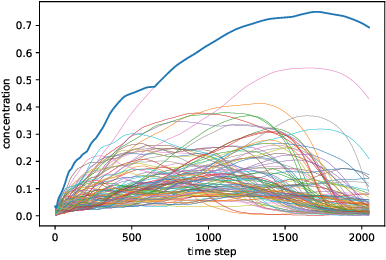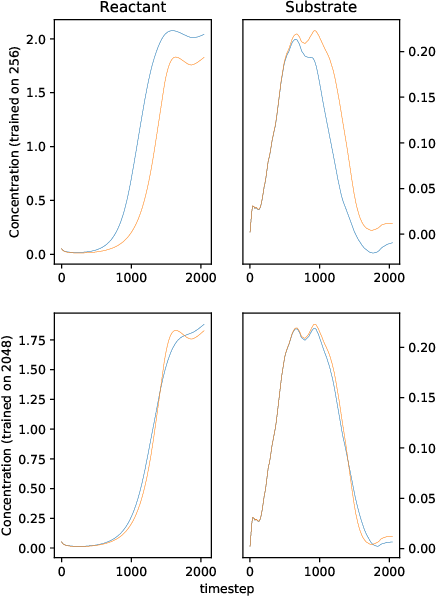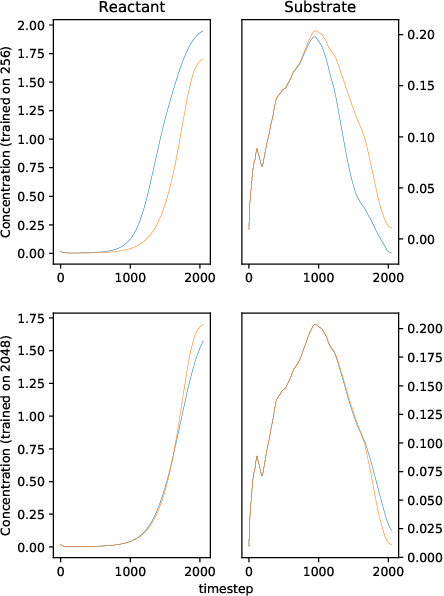Research papers and code for "Tobias Hagge":
Generative Adversarial Networks (GANs) are becoming popular choices for unsupervised learning. At the same time there is a concerted effort in the machine learning community to expand the range of tasks in which learning can be applied as well as to utilize methods from other disciplines to accelerate learning. With this in mind, in the current work we suggest ways to enforce given constraints in the output of a GAN both for interpolation and extrapolation. The two cases need to be treated differently. For the case of interpolation, the incorporation of constraints is built into the training of the GAN. The incorporation of the constraints respects the primary game-theoretic setup of a GAN so it can be combined with existing algorithms. However, it can exacerbate the problem of instability during training that is well-known for GANs. We suggest adding small noise to the constraints as a simple remedy that has performed well in our numerical experiments. The case of extrapolation (prediction) is more involved. First, we employ a modified interpolation training process that uses noisy data but does not necessarily enforce the constraints during training. Second, the resulting modified interpolator is used for extrapolation where the constraints are enforced after each step through projection on the space of constraints.

* 29 pages
Click to Read Paper and Get CodeWe solve a system of ordinary differential equations with an unknown functional form of a sink (reaction rate) term. We assume that the measurements (time series) of state variables are partially available, and we use recurrent neural network to "learn" the reaction rate from this data. This is achieved by including a discretized ordinary differential equations as part of a recurrent neural network training problem. We extend TensorFlow's recurrent neural network architecture to create a simple but scalable and effective solver for the unknown functions, and apply it to a fedbatch bioreactor simulation problem. Use of techniques from recent deep learning literature enables training of functions with behavior manifesting over thousands of time steps. Our networks are structurally similar to recurrent neural networks, but differences in design and function require modifications to the conventional wisdom about training such networks.

* 19 pages, 8 figures
Click to Read Paper and Get Code